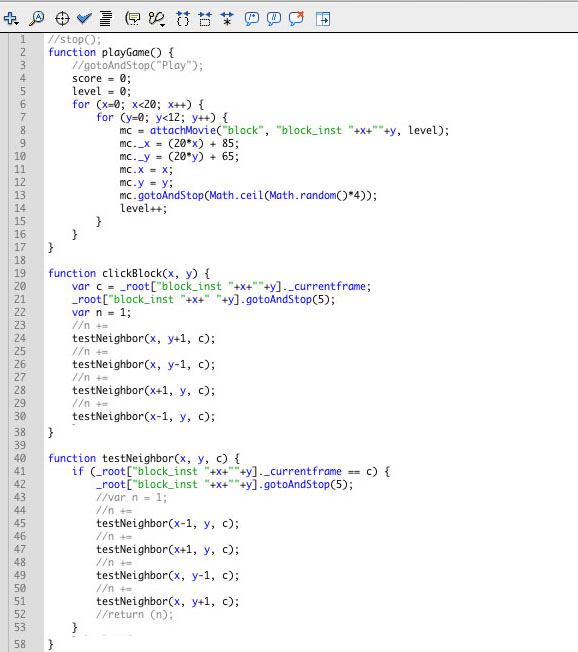Logout

5.1.2

Identify recursive thinking in a specified problem solution.

Teaching Note:

Sample Question:

sdfsdfsf

JSR Notes:

So here, you would be given a real-life or programming situation, and be asked to spot the recursive solution - which, at one level is pretty easy; just look for another instance of a particular method being called within one of the same name.

Example 1: As your first example, look back to 5.1.1 and the Little Old Lady code.

Example 2: And the following of recursion in Flash, with a scripting language you are probably not familiar with. But you can, simply, identify it as recursion, because there are calls to the testNeibhbor() method within the testNeighbor() method.

### Flash Game Recursion Example

Here's the game. (In 2020 Chrome still plays Flash files if you allow it.)

Here's the code:Example 3: The recursive binary search (explanation to follow in D.4.2 - this is just here so you can "identify" it is recursive.

```public static int recursiveBinarySearch(int[] arr, int low, int high, int key) {

if (low < high) {
int mid = low + high/ 2;
if (key < arr[mid]) {
return recursiveBinarySearch(arr, low, mid-1, key);  //<-- Ah ha!! Recursion!

} else if (key > arr[mid]) {
return recursiveBinarySearch(arr, mid+1, high , key); //<-- Ah ha!! Recursion!

} else {
return mid;
}
}
return -1;
}
```

Example 4: Factorial!

```public int factorial(int n) {
if(n == 1){
return 1;
}
else{
return n * factorial(n - 1);  //<--- Ah ha!! Recursion!
}
}

```

Example 5: Traversing a binary search tree (explanation to follow in D.4.2 - this is also just here so you can "identify" it is recursive.

```private String inOrderString = "";
private String traverseInOrder(StudentNode, String 2){
if(p != null){
traverseInOrder(p.getLeft(), inOrderString);  //<--- Ah ha!! Recursion!
inOrderString += p.getValue().getName();
traverseInOrder(p.getRight(), inOrderString);  //<--- Ah ha!! Recursion!
}
return inOrderString;
}
```